ECE4253 Digital Communications Department of Electrical and Computer Engineering - University of New Brunswick, Fredericton, NB, Canada

# Error Correction and the Hamming Code

The use of simple parity allows detection of single bit errors in a received message. Correction of such errors requires more information, since the position of the bad bit must be identified if it is to be corrected. (If a bad bit can be found, then it can be corrected by simply complementing its value.) Correction is not possible with one parity bit since any bit error in any position produces exactly the same information - "bad parity".

If more bits are included with a message, and if those bits can be arranged such that different errored bits produce different error results, then bad bits could be identified. In a 7-bit message, there are seven possible single bit errors, so three error control bits could potentially specify not only that an error occured but also which bit caused the error.

Similarly, if a family of codewords is chosen such that the minimum distance between valid codewords is at least 3, then single bit error correction is possible. This distance approach is geometric while the above error-bit argument is algebraic.

Either of the above arguments serves to introduce the Hamming Code, a binary block code allowing correction of single bit errors.

# The (7,4) Hamming Code

Consider a 4-bit message which is to be transmitted as a 7-bit codeword by including three parity bits. In general, this would be called a (7,4) code.

Three even parity bits (P) are computed on different subsets of the four message bits (D) as shown below.

 7 6 5 4 3 2 1 D D D P D P P 7-BIT CODEWORD D - D - D - P (EVEN PARITY) D D - - D P - (EVEN PARITY) D D D P - - - (EVEN PARITY)

 The three parity bits (1,2,4) are related to the data bits (3,5,6,7) as shown at right. In this diagram, each overlapping circle corresponds to one parity bit; each circle encompasses a different group of four bits and each circle is to have even parity. For example, parity bit 2 is computed so that its circle including data bits (3,6,7) is even parity. Given four data bits, the three parity bits can easily be chosen to ensure this condition. It can be observed that changing any one bit numbered 1..7 uniquely affects the three parity bits. Changing bit 7 affects all three parity bits, while an error in bit 6 affects only parity bits 2 and 4, and an error in a parity bit affects only that bit. The location of any single bit error can be established by rechecking the three parity circles.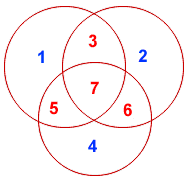For example, the message 1101 would be sent as 1100110, since:

 7 6 5 4 3 2 1 1 1 0 0 1 1 0 7-BIT CODEWORD 1 - 0 - 1 - 0 (EVEN PARITY) 1 1 - - 1 1 - (EVEN PARITY) 1 1 0 0 - - - (EVEN PARITY)

When these seven bits are entered into the parity circles, it can be confirmed that the choice of these three parity bits ensures that the parity within each circle is even, as shown here.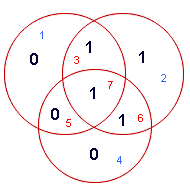It may now be observed that if an error occurs in any of the seven bits, that error will affect different combinations of the three parity bits depending on the bit position.

For example, suppose the above message 1100110 is sent and a single bit error occurs such that the codeword 1110110 is received:

```      transmitted message                           received message
1 1 0 0 1 1 0           ------------>          1 1 1 0 1 1 0
BIT:  7 6 5 4 3 2 1                            BIT:  7 6 5 4 3 2 1
```

The above error (in bit 5) can be corrected by examining which of the three parity bits was affected by the bad bit:

 7 6 5 4 3 2 1 1 1 1 0 1 1 0 7-BIT CODEWORD 1 - 1 - 1 - 0 (EVEN PARITY) NOT! 1 1 1 - - 1 1 - (EVEN PARITY) OK! 0 1 1 1 0 - - - (EVEN PARITY) NOT! 1

In fact, the bad parity bits labelled 101 point directly to the bad bit since 101 binary equals 5. Examination of the 'parity circles' confirms that any single bit error could be corrected in this way.

The value of the (7,4) Hamming code for error correction can be summarized:

1. Correction of single bit errors;
2. Cost of 3 bits added to a 4-bit message.

The ability to correct single bit errors comes at a cost which is less than sending the entire message twice. (Recall that simply sending a message twice does not allow error correction.)

# Complete Set of (7,4) Codewords

The complete set of codewords for the (7,4) Hamming Code code is shown below. A (7,4) code essentially defines 16 valid codewords from among 128 possible codewords. These sixteen codewords are special in that the distance between any two codewords is at least d=3.

 7 6 5 4 3 2 1 Hamming Code Generator - This circuit generates the (7,4) codewords at left from the four inputs bits (7,6,5,3) at the top. The XOR gates accomplish modulus 2 addition to determine the parity bits (4,2,1).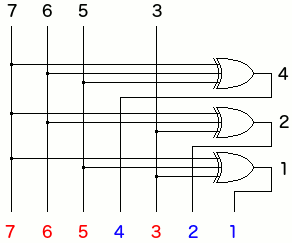0 0 0 0 0 0 0 0 1 0 0 0 0 1 1 1 2 0 0 1 1 0 0 1 3 0 0 1 1 1 1 0 4 0 1 0 1 0 1 0 5 0 1 0 1 1 0 1 6 0 1 1 0 0 1 1 7 0 1 1 0 1 0 0 8 1 0 0 1 0 1 1 9 1 0 0 1 1 0 0 A 1 0 1 0 0 1 0 B 1 0 1 0 1 0 1 C 1 1 0 0 0 0 1 D 1 1 0 0 1 1 0 E 1 1 1 1 0 0 0 F 1 1 1 1 1 1 1

The Hamming code is an example of a linear code because if any two of the above codewords are added together, the sum is another codeword.

# Hardware Error Correction

The circuit below checks and corrects a (7,4) Hamming codeword as shown above. The three bits (P4,P2,P1) represent the parity of the three circles in the Venn diagram; these will all be 0 (even parity) if no errors are detected. These same bits connect to a 3-of-8 decoder where a single output is active high depending on the state of the parity check (P4,P2,P1) inputs. If a bad input bit is detected, one non-zero decoder output will be high and the corresponding XOR gate will invert the bit that is in error. Any of the seven input bits could be corrected in this way; in practice, the circuit only needs to output the four data bits (7,6,5,3).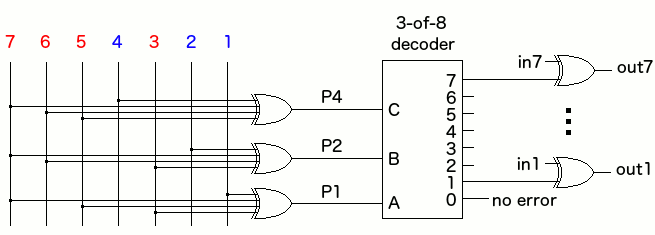This circuit does automatic checking and correction of single bit errors in the (7,4) Hamming code.

# The Distance Argument

 Looking again at the Venn diagram (at right) it can be observed that a change in any of the data bits (3,5,6,7) necessary changes at least two other bits in the codeword. For example, given a valid Hamming codeword, a change in bit 3 changes three bits (1,2,3) such that the new codeword is a distance (d=3) from the initial word. The clever arrangement of the Hamming codewords ensures that this is the case for every valid codeword in the set. Having distance (d=3) allows correction of single bit errors OR detection of 2-bit errors (because the two cases cannot be distinguished).# The (8,4) Extended Hamming Code

 If a 2-bit error occurs, the (7,4) Hamming code will interpret the event as an apparent single-bit error to be corrected. If an additional parity bit (P) is appended to the Hamming code as shown in the diagram at right, the resulting (8,4) codewords in the Extended Hamming Code will have distance (d=4). The new check bit (P) is computed as the even parity of the entire 7-bit Hamming code and improves the performance of the Hamming code whenever two bit errors occur. Having distance (d=4) allows correction of single bit errors and detection of 2-bit errors. Specifically, if a 1 bit error occurs, parity check (P) fails, while a 2-bit error is distinguished by the fact that parity check (P) passes.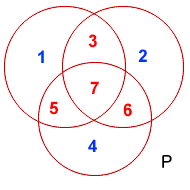# The (15,11) Hamming Code

The Hamming Code concept can be used for longer data words by including additional parity bits. Specifically, if four parity bits (8,4,2,1) are incorporated into a 15-bit codeword, single bit error correction is possible in the same way as the (7,4) code. In this case, the three overlapping circles would be become four overlapping spheres.

# Conclusions

Any set of codewords is useful for error control provided that the minimum distance between any two of them is some value D. (some may be more that D but none will be less than D)

There is no unique set of codewords with L=7 and D=3. The Hamming code shown here (L=7,D=3) is useful because it is easy to generate and to check this particular set of codewords by hand using the Venn diagram. The distance D=3 would still be achieved if two columns were swapped in the codewords or if the bits in any column were complemented, but the codewords would look very different (and the Venn diagrams could not be used).

Practical applications of the Hamming code use matrix methods to create codewords and to check for errors. See Hamming Code with Matrices

To examine sets of codewords with various distance properties, Online Codeword Generating Tool

The value of carefully choosing error control schemes is demonstrated by the (7,4) Hamming Code where set of codewords separated by a minimum distance D=3 allow for single-bit error correction.

 Sun Jul 3 21:25:30 ADT 2022 Last Updated: 26 JAN 2016 Richard Tervo [ tervo@unb.ca ]